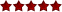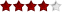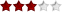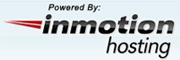Categories

1

DHTML CalculatorThis JavaScript implements a Calculator, which supports error checking. This DHTML Calculator. Performs functions like addition, subtraction, multiplication division etc like any other calculator.

Cost: Free

Bandwidth calculatorThis JavaScript absolves the problem in comprehending the various units used in calculating bandwidth. All it wants you to do is enter the bandwidth number and choose the input unit. This JavaScript returns the result in Bytes, Kilobytes, Megabytes and Gigabytes.

Cost: Free

Javascript Horsepower CalculatorThis JavaScript calculates the Horsepower for Cars. All it needs is the weight of the car, a timeslip, which indicates the time taken by the car to cross the quarter mile and speed of the car at the end of the quarter mile.

Cost: Free

Version: 1.0

4-digit Chmod calculatorThis JavaScript can be used to calculate and display the numeric value of permissions enabling you to correctly specify permission on your files. Displays the permission as Read, Write or Execute and its values.

Cost: Free

The Ratio GeneratorThis JavaScript works in a multiplicative inverse process and calculates the accurate fraction of any positive decimal number supplied. This can generate both proper and improper and also mixed fractions.

Cost: Freeware

OS: IE, NS

Solve EquationsThis program is used for calculating equations like, quadratic equation, cubic and quartic. Easy to handle and very easy to use.

Cost: Free

RPNcalculatorCompact RPN calculator with 99 stacks, trigonometric functions and userdefined variables. Runs in browsers supporting javascript and can be opened in popup windows. Can be handled completely using your keyboard and so enables a minimal size.

Cost: Free (GPL)

Version: 1.0

BiorhythmsEverything that happens around us in the nature is periodic.

Cost: free

EuroConverterThis is a JavaScript that converts any of the European currencies. The result are displayed even without clicking in Internet Explorer and in Netscape the result will be displayed after a simple click on the value of the currency.

Cost: Freeware

Version: 1.0

Byte ConverterThis JavaScript has been designed to work only in the Internet Explorer and can converts between bytes, kilobytes, megabytes and gigabytes. Displays different cells in the form of a table for each unit and allows customization of font, size, cell spacing etc.

Cost: Free

Version: 1.0

1

Need Scripts Message

We at Need Scripts, help You For Free, You can help us to serve you even better!General Web Hosting

Platform Based Hosting

Programmer's Hosting

Special Need's Hosting

Web Hosting Resources